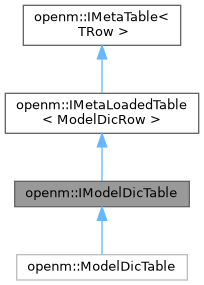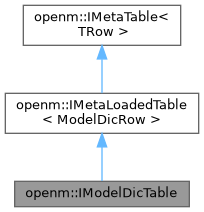OpenM++ runtime library (libopenm)
openm::IModelDicTable Struct Referenceabstract

model_dic table public interface. More...

Inheritance diagram for openm::IModelDicTable:[legend]
Collaboration diagram for openm::IModelDicTable:[legend]

## Public Member Functions

virtual const ModelDicRowbyKey (int i_modelId) const =0
binary search row by primary key: model id, return NULL if not found.

virtual const ModelDicRowbyNameDigest (const string &i_name, const string &i_digest) const =0
get find first row by model name and digest or NULL if not found.

virtual IRowBaseVecrowsRef (void)=0
get reference to list of all table rows.Public Member Functions inherited from openm::IMetaLoadedTable< ModelDicRow >
virtual const IRowBaseVecrowsCRef (void) const=0
get const reference to list of all table rows.

IRowBaseVec::difference_type rowCount (void) const
return number of rows.

vector< ModelDicRowrows (void) const
get list of loaded table rows.

const ModelDicRowfirstRow (void) const
return first table row or NULL if table is empty.

vector< ModelDicRowfindAll (RowEqual i_cmp) const
get list of rows by predicate: all rows where comparator to i_row return true.

const ModelDicRowfindFirst (RowEqual i_cmp) const
get first row by predicate or NULL if not found: first row where comparator to i_row return true.

const ModelDicRowbyIndex (IRowBaseVec::difference_type i_index) const
return row value by index or NULL if out of range.

IRowBaseVec::difference_type indexOf (RowEqual i_cmp, IRowBaseVec::difference_type i_startPos=0) const
get first row by predicate or -1 if not found: first row where comparator to i_row return true.

IRowBaseVec::size_type countOf (RowEqual i_cmp) const
count rows by predicate: where comparator to i_row return true.

## Static Public Member Functions

static IModelDicTablecreate (IDbExec *i_dbExec, const char *i_name=nullptr, const char *i_digest=nullptr)
create new table object and load table rows sorted by primary key: model id. More...

static IModelDicTablecreate (IDbExec *i_dbExec, int i_modelId)
create new table object and load table row by primary key: model id.

static IModelDicTablecreate (IRowBaseVec &io_rowVec)
create new table rows by swap with supplied vector of rows.Public Types inherited from openm::IMetaLoadedTable< ModelDicRow >
typedef function< bool(const ModelDicRow &i_dbRow)> RowEqual
db table row comparator.Protected Member Functions inherited from openm::IMetaLoadedTable< ModelDicRow >
const ModelDicRowfindKey (const IRowBaseUptr &i_row) constStatic Protected Member Functions inherited from openm::IMetaLoadedTable< ModelDicRow >
load table: return vector of selected rows sorted by primary key.Static Protected Member Functions inherited from openm::IMetaTable< TRow >
static vector< TRow > rows (const IRowBaseVec &i_rowVec)
get list of table rows.

## Detailed Description

model_dic table public interface.

## ◆ create()

 IModelDicTable * IModelDicTable::create ( IDbExec * i_dbExec, const char * i_name = `nullptr`, const char * i_digest = `nullptr` )
static

create new table object and load table rows sorted by primary key: model id.

if name and/or digest specified then select only rows where model_name = i_name and model_digest = i_digest

The documentation for this struct was generated from the following files: